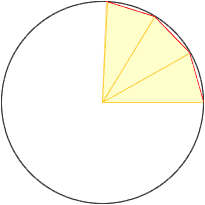Name: Sophie Level: Secondary I am a student We have been given a piece of maths coursework. A farmer has exactly 1000 metres of fencing and wants to fence off a plot of level land. she does not mind what the shape is but it must have a perimeter of 1000m. She wants to fence off the plot of land which contains the maximum area. Investigate the shape, or shapes that could be used to fence in the maximum area using exactly 1000 metres of fencing each time. I have investigated many shapes, and I feel that a circle will have the biggest area. However we have also been asked to investigate shapes with 20 and 30 sides. My dad said that there is a formula for finding out any area of land. Do you know of this formula, if so I would be very grateful if you were to email it to me. Yours faithfully Sophie Hi Sophie, I expect that by "shapes with 20 and 30 sides" what was meant was regular polygons with 20 and 30 sides. I don't carry a formula in my head but I can show you how to do it. To construct a regular n-gon (a regular polygon with n sides) draw a circle and construct the n-gon inside the circle. (I started to construct a 12-gon in the diagram below.)Th n-gon is composed of n iscosceles triangles, each with one angle of  360/n degrees. The length of each of the two equal sides is the radius of the circle, r, and the length of the other side is  1000/n. Let h be the height of the triangle as in the diagram below.The angle t in the diagram is  360/2n degrees and the tangent of t is  500/nh. Hence the area of the triangle is  500/n times the tangent of  360/2n degrees. Once you have the area of the triangle, multiply by n to get the area of the n-gon. I hope this helps, Penny Go to Math Central To return to the previous page use your browser's back button.